# scikit-learn/scikit-learn

Have a question about this project? Sign up for a free GitHub account to open an issue and contact its maintainers and the community.

By clicking “Sign up for GitHub”, you agree to our terms of service and privacy statement. We’ll occasionally send you account related emails.

# Fixes #13173, implements faster polynomial features for dense matrices #13290

Open
wants to merge 15 commits into
from

## Conversation

Projects

### sdpython commented Feb 26, 2019

Fixes #13173.

#### What does this implement/fix? Explain your changes.

This PR implements a faster version of polynomial features for dense matrices. Instead of computing each features independently by going through all combinations, it broadcasts as much as possible the multiple by one vector. The speed up is significant (3 times faster) for matrices with less than 10.000 rows.

#### Any other comments?

More details about speed up can be found here: https://github.com/sdpython/mlinsights/blob/master/_doc/notebooks/sklearn/faster_polynomial_features.ipynb.

``` Fixes #13173, implements faster polynomial features for dense matrices ```
``` 670b0f1 ```

### sdpython added some commits Feb 26, 2019

``` fix a bug occuring when interation_only is True ```
``` ae847ce ```
``` fixes interactive_only ```
``` 3d81e4a ```
``` pep8 ```
``` e19ccb0 ```
``` pep8 ```
``` 607dd9a ```
Member

### GaelVaroquaux commented Feb 27, 2019

 Can you add the benchmark script that you used in the benchmarks folder of the repo and paste here the graph that it outputs.

### sdpython added some commits Feb 27, 2019

``` Merge branch 'master' of https://github.com/scikit-learn/scikit-learn … ```
`…into poly`
``` 833f0d9 ```
``` Add script to benchmark implementations ```
``` ed8585d ```
Author

### sdpython commented Feb 27, 2019 • edited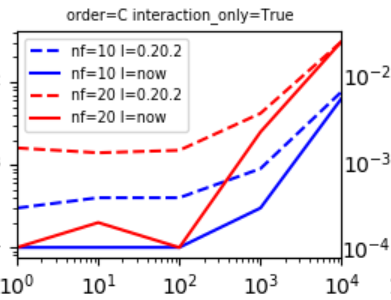X-axis is number of observations Y-axis is time in seconds ``````degree interaction_only n nfeat order time_0_20_2 time_current `````` 1 2 True 1 10 C 0.000299 0.000100 25 2 True 10 10 C 0.000399 0.000100 49 2 True 100 10 C 0.000399 0.000100 73 2 True 1000 10 C 0.000898 0.000299 97 2 True 10000 10 C 0.007676 0.006383 degree interaction_only n nfeat order time_0_20_2 time_current 13 2 True 1 20 C 0.001596 0.000100 37 2 True 10 20 C 0.001396 0.000199 61 2 True 100 20 C 0.001496 0.000100 85 2 True 1000 20 C 0.004189 0.002495 109 2 True 10000 20 C 0.031014 0.031117
Member

### ogrisel commented Feb 27, 2019

 The benchmark script needs a couple of quick fixes: ``````benchmarks/bench_plot_polynomial_features.py:10:1: F401 'sys' imported but unused import sys ^ benchmarks/bench_plot_polynomial_features.py:11:1: F401 'warnings' imported but unused import warnings ^ benchmarks/bench_plot_polynomial_features.py:12:1: F401 'numbers' imported but unused import numbers ^ benchmarks/bench_plot_polynomial_features.py:24:1: F401 'sklearn.utils.testing.ignore_warnings' imported but unused from sklearn.utils.testing import ignore_warnings ^ benchmarks/bench_plot_polynomial_features.py:87:80: E501 line too long (94 > 79 characters) degree, interaction_only, order) ^ benchmarks/bench_plot_polynomial_features.py:89:80: E501 line too long (87 > 79 characters) degree, interaction_only, order) ^ benchmarks/bench_plot_polynomial_features.py:105:38: E261 at least two spaces before inline comment break # stops if longer than a second ``````
Member

### ogrisel commented Feb 27, 2019

 Can you please do a benchmark for a larger number of features? The new code is significantly more complex than the old one so I would like to see case where the new code is significantly faster than the old code on a case that lasts more than 10s.
Member

### ogrisel commented Feb 27, 2019

 Arguably it might still be interesting to be significantly faster on a small number of samples at prediction time to reduce the single sample prediction latency but I am not sure that a change from 0.3ms to 0.1ms is important enough to justify the increase in code complexity.

### sdpython added some commits Feb 27, 2019

``` pep8 ```
``` 12369a8 ```
``` Update bench_plot_polynomial_features.py ```
``` 91936cf ```
Author

### sdpython commented Feb 28, 2019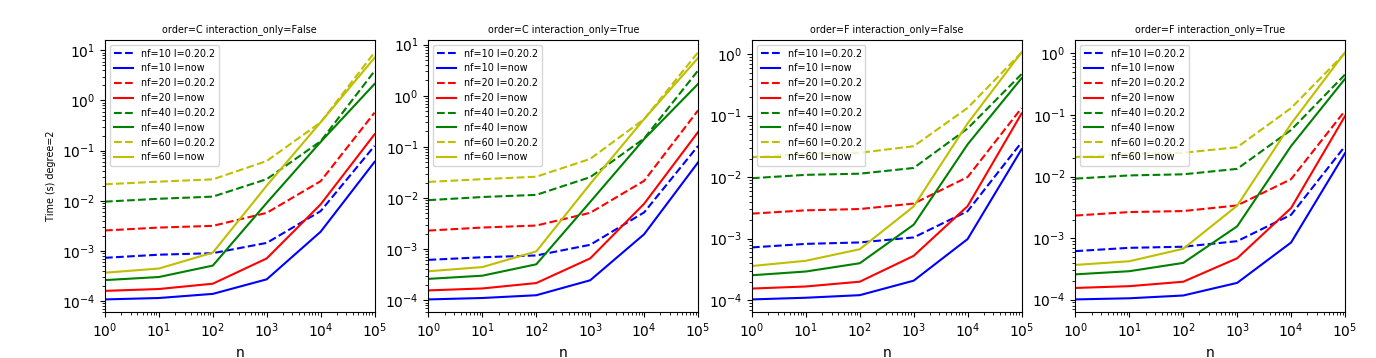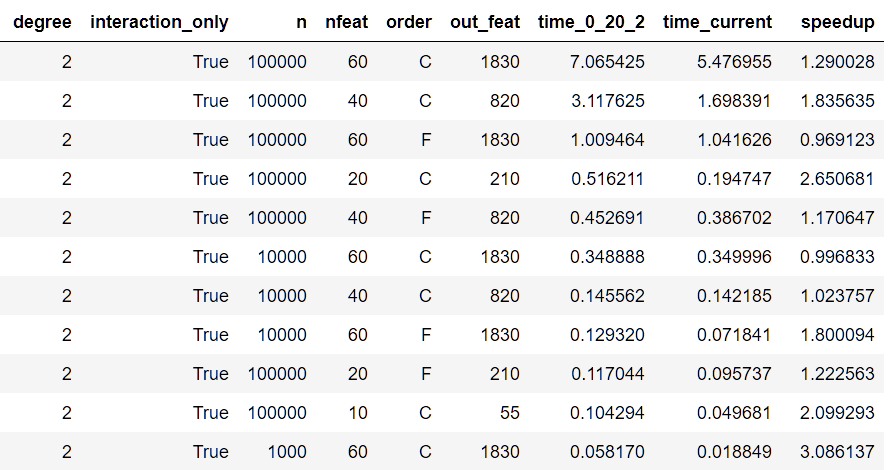You are probably right about the speed up. However, the new version is a better inspiration for anybody who needs to implement a runtime for fast predictions. That was also one of my objectives by proposing this change. I don't know if many people search for numerical recipes inside scikit-learn code. I do that sometimes.
Member

### ogrisel commented Feb 28, 2019 • edited

 Thank you for the additional benchmark results. So indeed for a large number of features the difference starts to be significant. Maybe this code a good candidate for cythonization but I am not saying we should wait for cythonization to merge this PR. I would like to have other people opinions in the complexity vs performance tradeoff of this PR.

### sdpython added some commits Feb 28, 2019

``` Merge branch 'master' of https://github.com/scikit-learn/scikit-learn … ```
`…into poly`
``` a36c581 ```
``` reduce the number of data copies, improves memory footprints ```
``` 71ae492 ```
Author

### sdpython commented Feb 28, 2019 • edited

 I just made a fix and remove one data copy (no copy of np.multiply result) and the improvment is significant even for 100.000 rows.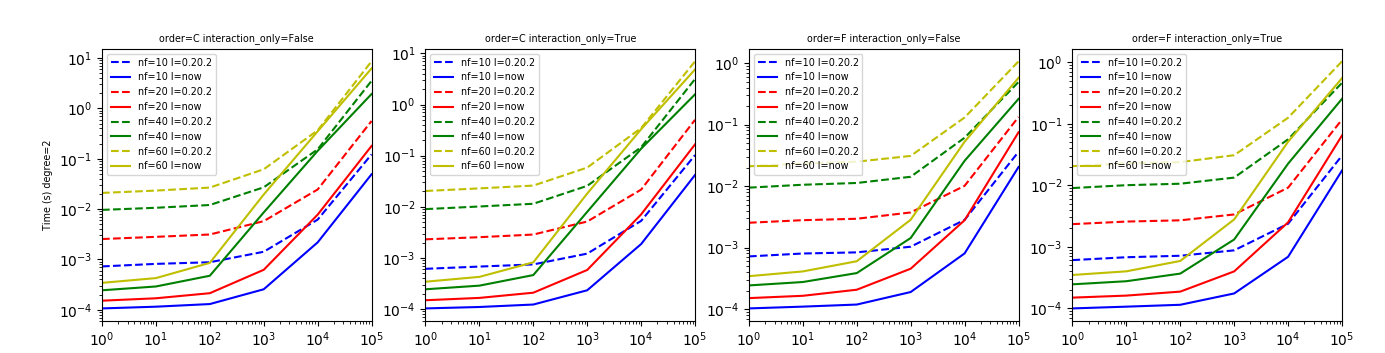Basically, the implementation is almost twice faster than the current one for matrices with 100.000 rows.

### jeremiedbb reviewed Feb 28, 2019

 # the matrix first to multiply contiguous memory segments. transpose = X.size <= 1e7 and self.order == 'C' n = X.shape

#### jeremiedbb Feb 28, 2019

Contributor

When the size is larger, is transposing slower or equivalent ?
And, when the matrix is small does the transposing bring a significant part of the speed up ?

My point is that the transpose switch makes the code twice as long, and I'm wondering if we could always do one or the other.

#### sdpython Feb 28, 2019

Author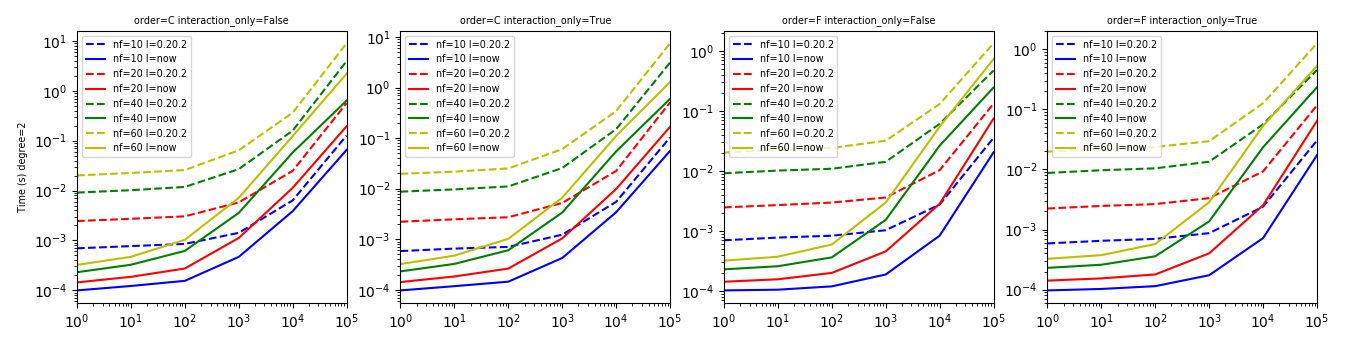I remove the transpose and it seemed better. So i'll keep it. I added it because it was better before I removed one unnecessary copy this morning. transpose is inefficient when order=='F' so the only option to simplify is to remove it. Updating the PR.

``` remove transpose ```
``` ee646a5 ```
Member

### GaelVaroquaux commented Feb 28, 2019

 We are trying to evaluate whether the speedups in this PR are important or not for application (because they are a constant offset, and not a proportional benefit. We came up with a potentially useful situation: in an online learning setting, where the polynomial features are applied on minibatches (typically of size 100), which are then fed into an SGDClassifier with "partial_fit". The question is then: what is the relative cost of on call to the partial_fit of SGDClassifier versus the cost of the transform of the polynomial features. Could you run a benchmark on such an example? Thanks!
Author

### sdpython commented Feb 28, 2019 • edited

 Hi, I created a benchmark which tests the four following functions: ``````def fcts_model(X, y): model1 = SGDClassifier() model2 = make_pipeline(PolynomialFeatures(), SGDClassifier()) model3 = make_pipeline(ExtendedFeatures(kind='poly'), SGDClassifier()) model4 = make_pipeline(ExtendedFeatures(kind='poly-slow'), SGDClassifier()) model1.fit(PolynomialFeatures().fit_transform(X), y) model2.fit(X, y) model3.fit(X, y) model4.fit(X, y) def partial_fit_model1(X, y, model=model1): return model.partial_fit(X, y) def partial_fit_model2(X, y, model=model2): X2 = model.steps.transform(X) return model.steps.partial_fit(X2, y) def partial_fit_model3(X, y, model=model3): X2 = model.steps.transform(X) return model.steps.partial_fit(X2, y) def partial_fit_model4(X, y, model=model4): X2 = model.steps.transform(X) return model.steps.partial_fit(X2, y) return partial_fit_model1, partial_fit_model2, partial_fit_model3, partial_fit_model4 `````` It tests the combination of PolynomialFeatures + SGDClassifier. On the following graph: SGD = SGDClassifier only, SGD-SKL = SGD + POLY 0.20.2 , SGD-FAST is the new implementation, SGD-SLOW is 0.20.2 but implemented in the bench to make sure I'm not sure my branch to make the test. Is that what you expected?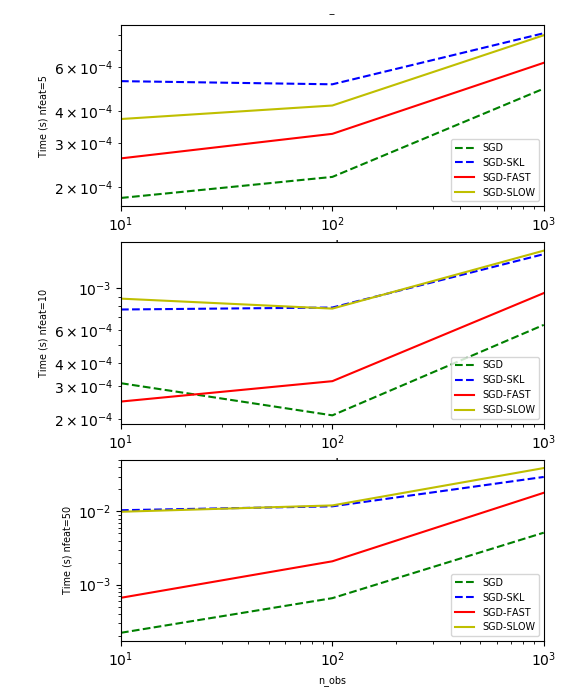Member

### ogrisel commented Feb 28, 2019

 It tests the combination of PolynomialFeatures + SGDClassifier. On the following graph: SGD = SGDClassifier only, SGD-SKL = SGD + POLY 0.20.2 , SGD-FAST is the new implementation, SGD-SLOW is 0.20.2 but implemented in the bench to make sure I'm not sure my branch to make the test. This is really confusing. Can you please rephrase?

Member

### ogrisel left a comment

sklearn/preprocessing/data.py Outdated
sklearn/preprocessing/data.py Outdated
 if self.interaction_only: start = a + index[i+1] - index[i] else: start = a

#### ogrisel Feb 28, 2019

Member

This could be simplified as:

```                            new_index.append(pos)
start = index[i]
if self.interaction_only:
start += index[i+1] - index[i]```

Author

Done

### sdpython added some commits Mar 1, 2019

``` Merge branch 'master' of https://github.com/scikit-learn/scikit-learn … ```
`…into poly`
``` 1a970de ```
``` Fix comment from PR + add second benchmark ```
``` d9a94d6 ```
Author

### sdpython commented Mar 1, 2019

 I added the second benchmark to the sources: SGD=SGDClasifier only SGD-SKL=PolynomialFeatures from scikit-learn (no matter what it is) SGD-FAST=new implementation copy-pasted in the benchmark source file SGD-SLOW=implementation of 0.20.2 copy-pasted in the benchmark source file
Member

### GaelVaroquaux commented Mar 1, 2019

 I hate to say, but I find the benchmark code very hard to read. It's very factored and generic. I would personally write much simpler code, even if it repeats a bit more.
Author

### sdpython commented Mar 2, 2019

 Both benchmarks I assume?
Member

### GaelVaroquaux commented Mar 2, 2019

 I had the online one in mind.
``` refactor second benchmark ```
``` b518147 ```
Author

### sdpython commented Mar 3, 2019

 I updated the second benchmark and the code. Below what I measure written in a different way. ``````def measure_sgd_only(X_train, y_train, Xs, ys): model = SGDClassifier() model.fit(PolynomialFeatures().fit_transform(X_train), y_train) Xs = [PolynomialFeatures().fit_transform(x) for x in Xs] st = time() for X, y in zip(Xs, ys): model.partial_fit(X, y) end = time() return end - st def measure_sgd_poly_skl(X_train, y_train, Xs, ys): model = make_pipeline(PolynomialFeatures(), SGDClassifier()) model.fit(X_train, y_train) st = time() for X, y in zip(Xs, ys): X2 = model.steps.transform(X) model.steps.partial_fit(X2, y) end = time() return end - st def measure_sgd_poly_fast(X_train, y_train, Xs, ys): model = make_pipeline(CustomPolynomialFeatures(kind='poly-fast'), SGDClassifier()) model.fit(X_train, y_train) st = time() for X, y in zip(Xs, ys): X2 = model.steps.transform(X) model.steps.partial_fit(X2, y) end = time() return end - st def measure_sgd_poly_slow(X_train, y_train, Xs, ys): model = make_pipeline(CustomPolynomialFeatures(kind='poly-slow'), SGDClassifier()) model.fit(X_train, y_train) st = time() for X, y in zip(Xs, ys): X2 = model.steps.transform(X) model.steps.partial_fit(X2, y) end = time() return end - st ``````
Author

### sdpython commented Mar 3, 2019 • edited

 I was looking for a way to show how the improvment works. The following graph plots the ratio (new execution time / execution time of 0.20.2) accross all configurations. This shows how it moves against the number of features and observations.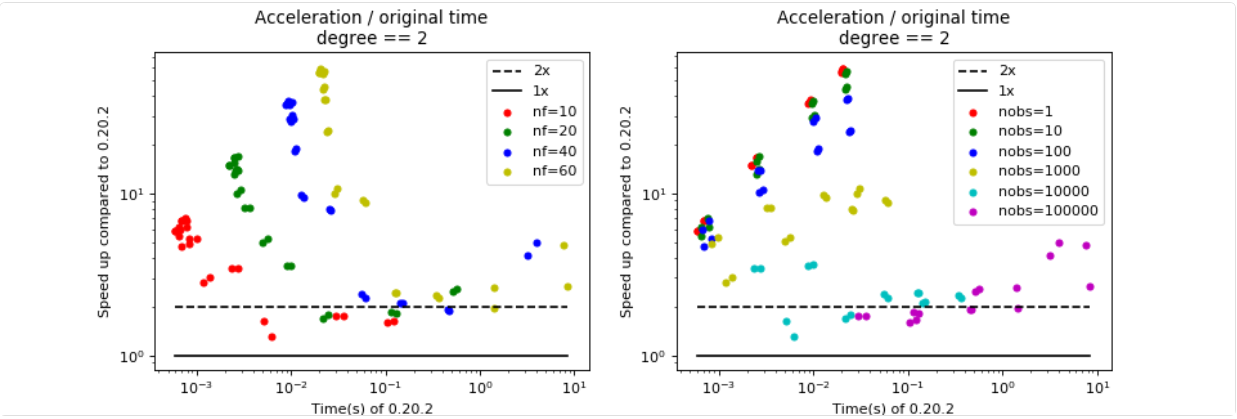to join this conversation on GitHub. Already have an account? Sign in to comment
You can’t perform that action at this time.